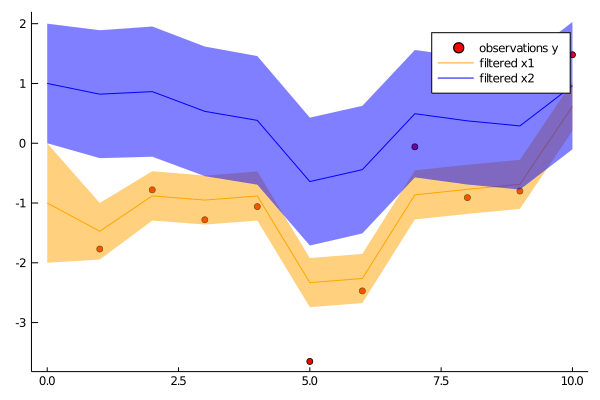## Kalman.jl

Flexible filtering and smoothing in Julia
Popularity
69 Stars
Updated Last
6 Months Ago
Started In
February 2018# Kalman

Flexible filtering and smoothing in Julia. `Kalman` uses `DynamicIterators` (an iterator protocol for dynamic data dependent and controlled processes) and `GaussianDistributions` (Gaussian distributions as abstraction for the uncertain state) to implement flexible online Kalman filtering.

The package provides tools to filter and smooth and conditionally sample the state space system

``````x[k] = Φx[k−1] + b + w[k],    w[k] ∼ N(0, Q)

y[k] = Hx[k] + v[k],    v[k] ∼ N(0, R)
``````

## How to use

One way, and maybe the way, to use this package is to use `Gaussian` from `GaussianDistributions.jl` as representation of mean and uncertainty of a filter and call `Kalman.correct` to implement the correction step in a Kalman filter:

```using Kalman, GaussianDistributions, LinearAlgebra
using GaussianDistributions: ⊕ # independent sum of Gaussian r.v.
using Statistics

# prior for time 0
x0 = [-1., 1.]
P0 = Matrix(1.0I, 2, 2)

# dynamics
Φ = [0.8 0.2; -0.1 0.8]
b = zeros(2)
Q = [0.2 0.0; 0.0 0.5]

# observation
H = [1.0 0.0]
R = Matrix(0.3I, 1, 1)

# (mock) data
ys = [[-1.77], [-0.78], [-1.28], [-1.06], [-3.65], [-2.47], [-0.06], [-0.91], [-0.80], [1.48]]

# filter (assuming first observation at time 1)
N = length(ys)

p = Gaussian(x0, P0)
ps = [p] # vector of filtered Gaussians
for i in 1:N
global p
# predict
p = Φ*p ⊕ Gaussian(zero(x0), Q) #same as Gaussian(Φ*p.μ, Φ*p.Σ*Φ' + Q)
# correct
p, yres, _ = Kalman.correct(Kalman.JosephForm(), p, (Gaussian(ys[i], R), H))
push!(ps, p) # save filtered density
end

using Plots

p1 = scatter(1:N, first.(ys), color="red", label="observations y")
plot!(p1, 0:N, [mean(p) for p in ps], color="orange", label="filtered x1", grid=false, ribbon=[sqrt(cov(p)[1,1]) for p in ps], fillalpha=.5)
plot!(p1, 0:N, [mean(p) for p in ps], color="blue", label="filtered x2", grid=false, ribbon=[sqrt(cov(p)[2,2]) for p in ps], fillalpha=.5)

savefig(p1, "filter.png")```A very similar example of tracking a 2D trajectory can be found here.

## Interface

The same might be achieved using interface functions

```using DynamicIterators, GaussianDistributions, Kalman, LinearAlgebra

# Define linear evolution
Φ = [0.8 0.5; -0.1 0.8]
b = zeros(2)
Q = [0.2 0.0; 0.0 1.0]

E = LinearEvolution(Φ, Gaussian(b, Q))

# Define observation scheme
H = [1.0 0.0]
R = Matrix(1.0I, 1, 1)

O = LinearObservation(E, H, R)

# Prior
x0 = [1., 0.]
P0 = Matrix(1.0I, 2, 2)

# Observations (mock)
Y = [1 => [1.14326], 2 => [-0.271804], 3 => [-0.00512675]]

# Filter
Xf, ll = kalmanfilter(O, 0 => Gaussian(x0, P0), Y)
@show Xf
```

## Implementation

As said, filtering is implemented via the DynamicIterator protocol. It is worthwhile to look at a possible the implementation of `kalmanfilter` to see how filtering can be integrated into online algorithms (run in a local scope to avoid `UndefVarError: ystate not defined`.)

```# `Y` is the data iterator, iterating over pairs of  `t => v` of time `t` and observation `v`
# `O` is the dynamical filter iterator, iterating over pairs `t => u` where
#     u::Tuple{<:Gaussian,<:Gaussian,Float64}
# is the tuple of filtered state, the predicted state and the log likelihood

# Initialise data iterator

ϕ = iterate(Y)
ϕ === nothing && error("no observations")
(t, v), ystate = ϕ

# Initialise dynamical filter with first data point `t => v`
# and the `prior::Pair{Int,<:Gaussian}`, a pair of initial time and initial state
prior = 0 => Gaussian(x0, P0)

ϕ = dyniterate(O, Start(Kalman.Filter(prior, 0.0)), t => v)
ϕ === nothing && error("no observations")
(t, u), state = ϕ

X = [t => u]
while true

ϕ = iterate(Y, ystate)
ϕ === nothing && break
(t, v), ystate = ϕ

# Advance filter with new data `t => v`

ϕ = dyniterate(O, state, t => v)
ϕ === nothing && break
(t, u), state = ϕ

# Do something with the result `t => u` (here: saving it)

push!(X, t => u) # save filtered state
end
ll = u # likelihood
@show  X, ll```

### Required Packages

View all packages

### Used By Packages

No packages found.